# ILP Part 43 — Maximum revisited

This is the forty thrid part of the ILP series. For your convenience you can find other parts in the table of contents in Part 1 – Boolean algebra

We have already seen how to implement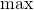function in ILP. Last time we used comparisons, today we will perform bit operations.

# Idea

Having non-negative variables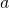and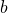we want to calculate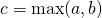. First, we decompose variables into its binary representation as in multiplication. Nest, we use the following trick: if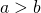then there must be a n indexfor which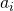is one and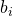is zero.

Proof: let’s assume thatwhich means that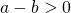, and there is no index for which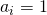and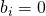. At the same time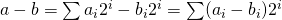. But since there is no index we want to find, each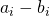is not greater than zero. So the whole sum is not greater than zero — contradiction.

Now we only need to find this index.

# Finding index

We will simply bruteforce index. For each position we define the following variables: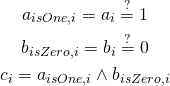Now,is desired index if and only if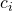is one and all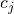for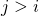is zero: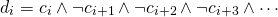The only missing bit: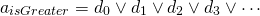Now we can perform multiplication to select maximum value using calculated flag.